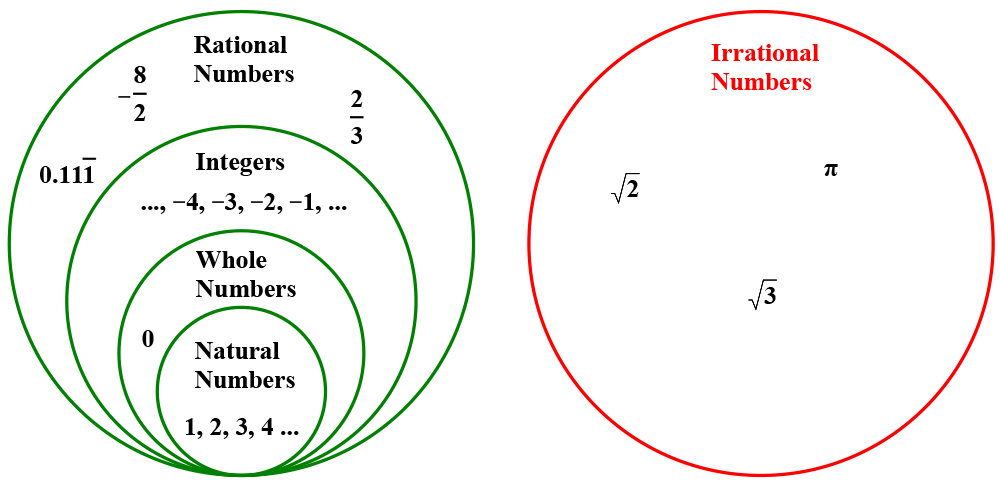Rational and Irrational numbers

I only recently properly considered why all fractions are either terminating or recurring decimals.  Fundamentally, this is because there are a finite number of options for the remainder, which is a maximum of 1 less than the divisor.  This is most easily seen when dividing by 7.  All six potential remainders are used and the 7th division goes back to the beginning of the sequence as shown on this task from Don Steward.Maybe this is an important thing to establish before talking about irrational numbers. With irrational numbers, we are effectively trying to convince students that there is a separate class of numbers on the number line that can’t be expressed as a division of two integers.There is a proof for why ∏ is irrational but it’s not pretty. I’m taking Peter Mitchell’s word for it on that who presented on this topic at the recent MEI conference.  He has a proof here, but in his own words “it’s really, really tedious!”  So maybe surds are a better place to look for an example of a proof that irrational numbers exist. Although this is an A level topic, I think with the right class this could be used at KS4.

Proof that √2 is irrational

This is a proof by contradiction, which in itself is a bit strange.  But the logic is sound: if I assume something to be true and then work through it to show that there is something inherent within it that is false, then I have proved that thing cannot be true therefore it must be false.

In this case, we are going assume that √2 is a rational number, prove that that is false, thereby proving that √2 must be irrational.

If √2 is a rational number, then we can write it √2  = a/b where ab are whole numbers, b not zero.

We additionally assume that this a/b is simplified to lowest terms, since that can obviously be done with any fraction. Notice that in order for a/b to be in simplest terms, both of a and b cannot be even. One or both must be odd. Otherwise, we could simplify a/b further.

Going back to our first statement:

√2  = a/b

we can square both sides to get:

2 = a2/b2

or

a2 = 2b2.

So the square of a must be an even number since it is two times something.   If is even then a itself must also be even. Any odd number time an odd number creates an odd number (some more of these here).

Okay, if a itself is an even number, then a is 2 times some other whole number. In symbols, a = 2k where k is this other number.

If we substitute a = 2k into the original equation 2 = a2/b2:

2 = (2k)2/b2

2 = 4k2/b2

2b2 = 4k2

b2 = 2k2

Again, because b is 2 times something, b must be an even number.

We have shown that a and b are both even numbers, but we started saying that a/b was a fraction in its simplest form.

I might want students to explore what happens with √4 in this same proof, i.e. prove why √4 is not irrational.  From there we could go on to look at √3.  It’s a bit harder, but only really requires that all odd numbers can be written in the form 2n+1.  Here is a spoiler if you are stuck.

Concentric Equilateral Triangles

The red equilateral triangle side length 4cm sits inside the larger pink equilateral triangle such that the “border” is 1cm wide.What is the ratio of the height of the red triangle to the height of the pink triangle?

Can you solve using trigonometry or only using Pythagoras?

The border is now 2cm, whilst the side length of the red triangle remains 4cm.  What is the ratio of heights now?Explore what happens for other border widths.  Can you generalise for any border width w?

Geogebra file here.

Spoiler here.

I got a few solutions posted on Twitter for this, but the most elegant (so far…) has to be this from @mathforge. As he said: no Trig, no Pythagoras, just ratio.Hippocrates First Theorem

Another one from the fabulous Don Steward:You could of course just go straight for the algebraic proof but it does require a level of confidence with surds.  So you might want to scaffold this task. Maybe start by putting some numbers in for the radius of the smaller semi-circle, maybe 2.  You could then do it again with 4 and ask students if they are convinced by that.  (Here are some examples to warn against the dangers of extrapolating what appears to be a pattern).  If you do take the numbers approach it’s good calculator practice.  Can you type the whole expression for the area of the curved shape into the calculator to get an exact answer?

And here is a little GeoGebra drawing to go with it.